Ex 6.2

Chapter 6 Class 6 Integers
Serial order wise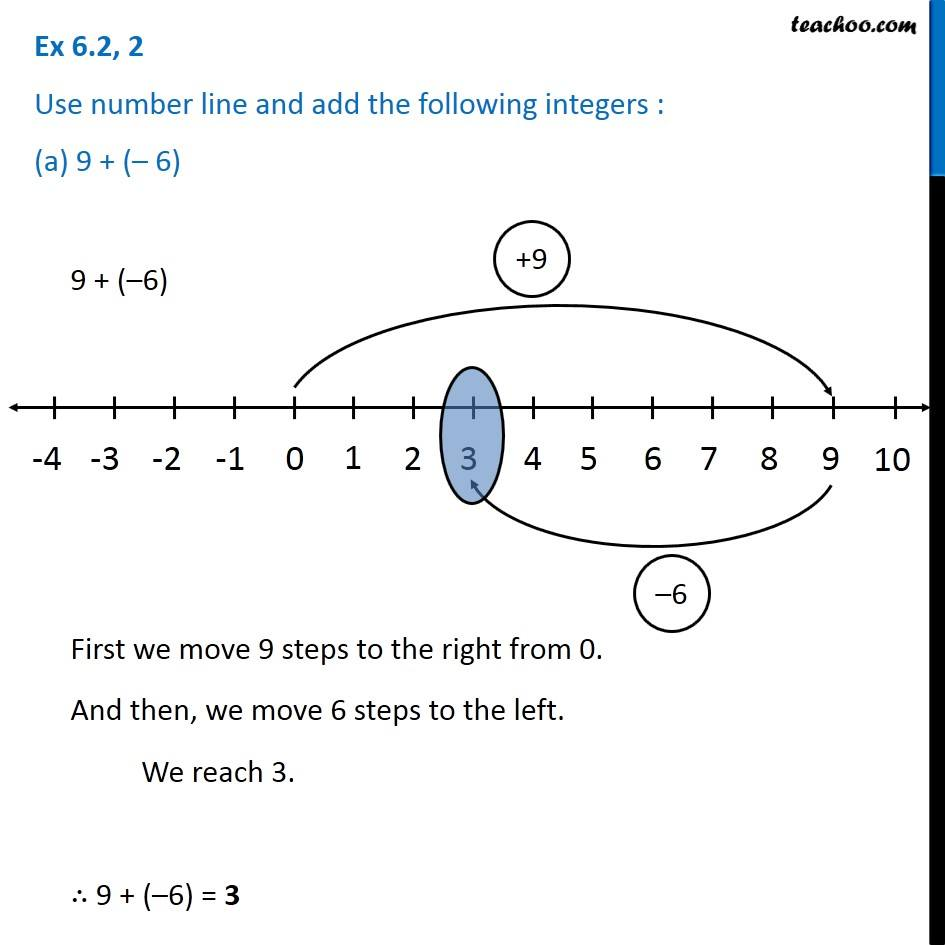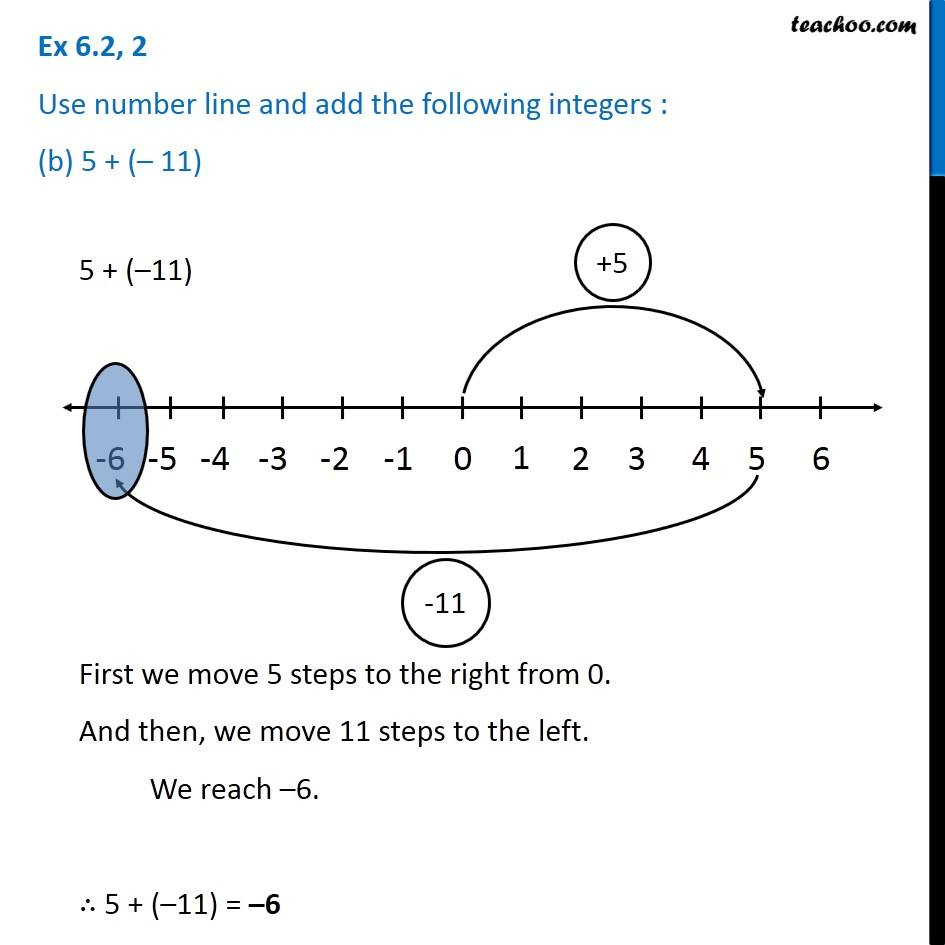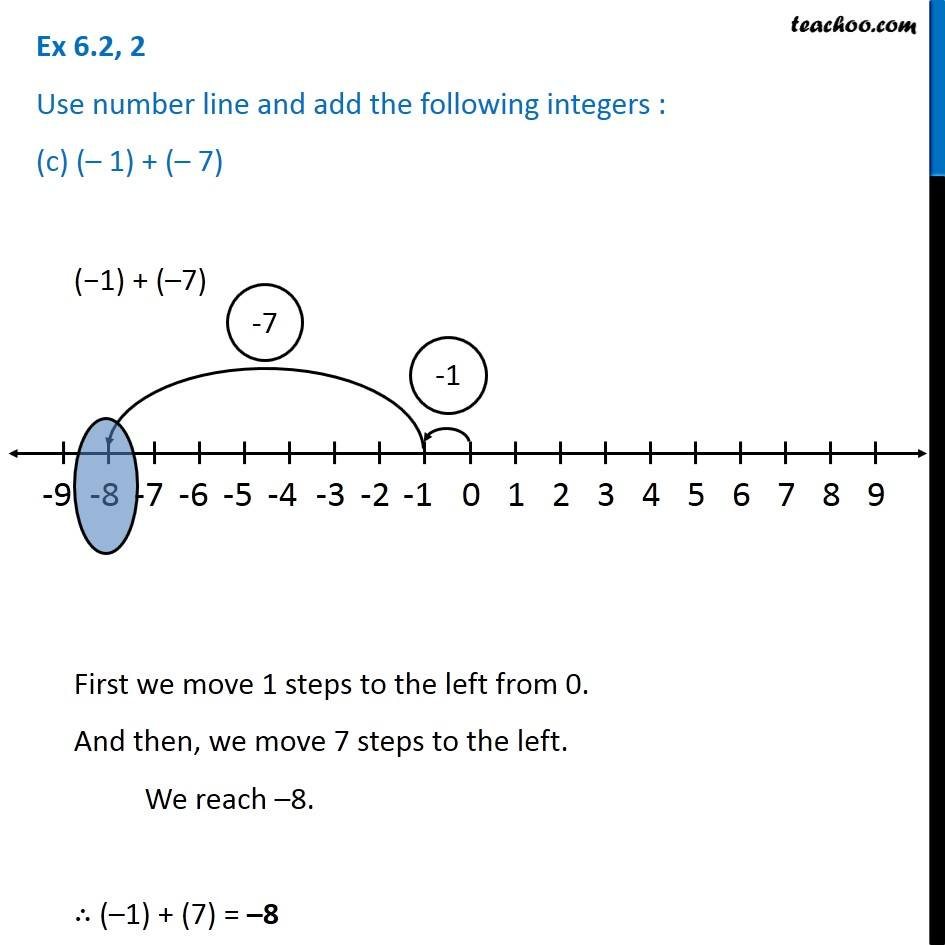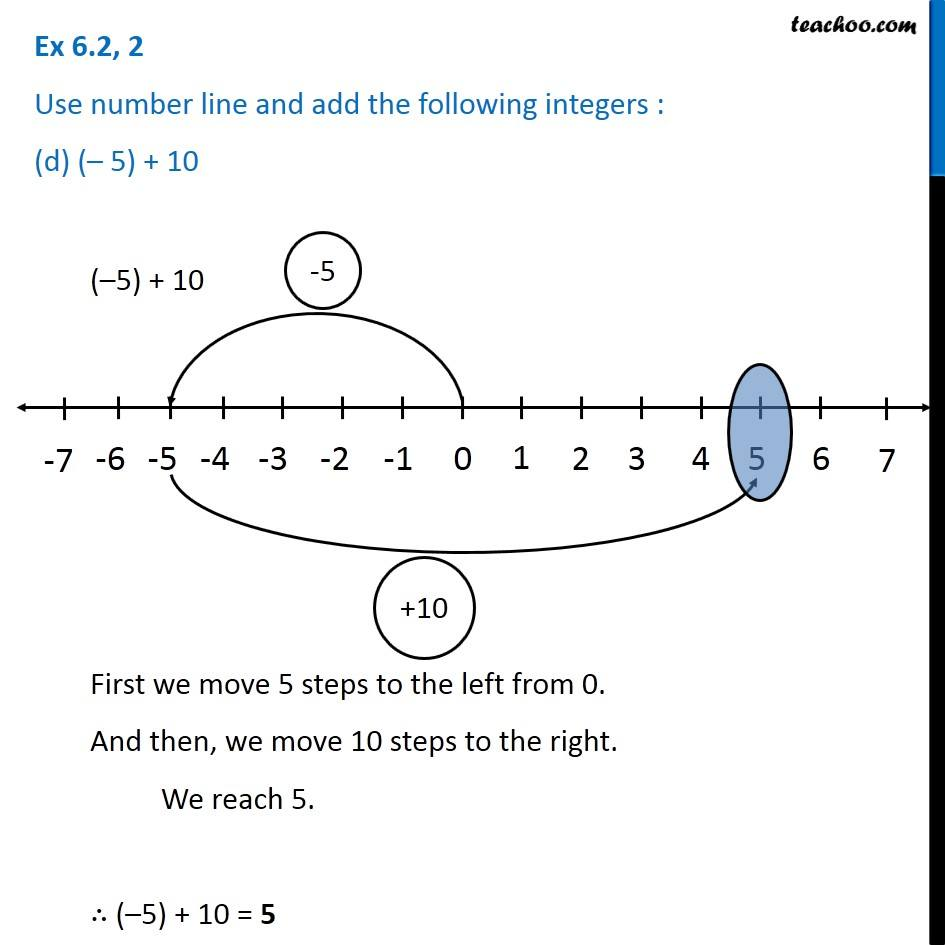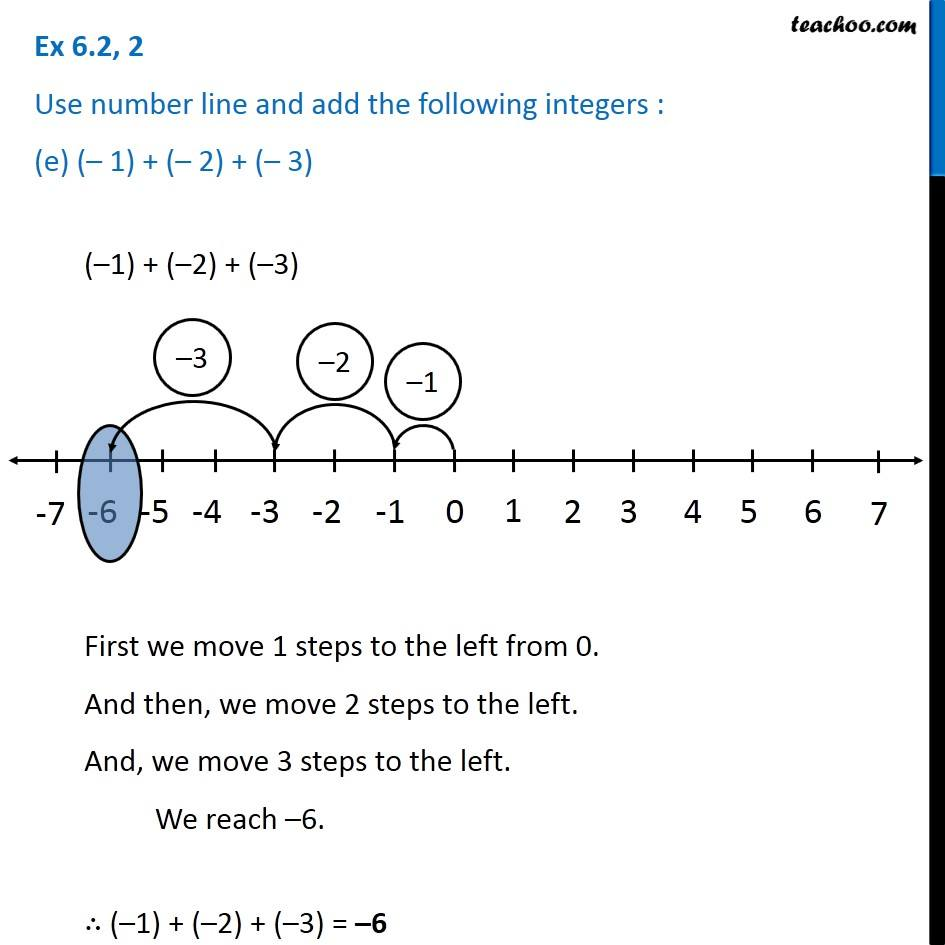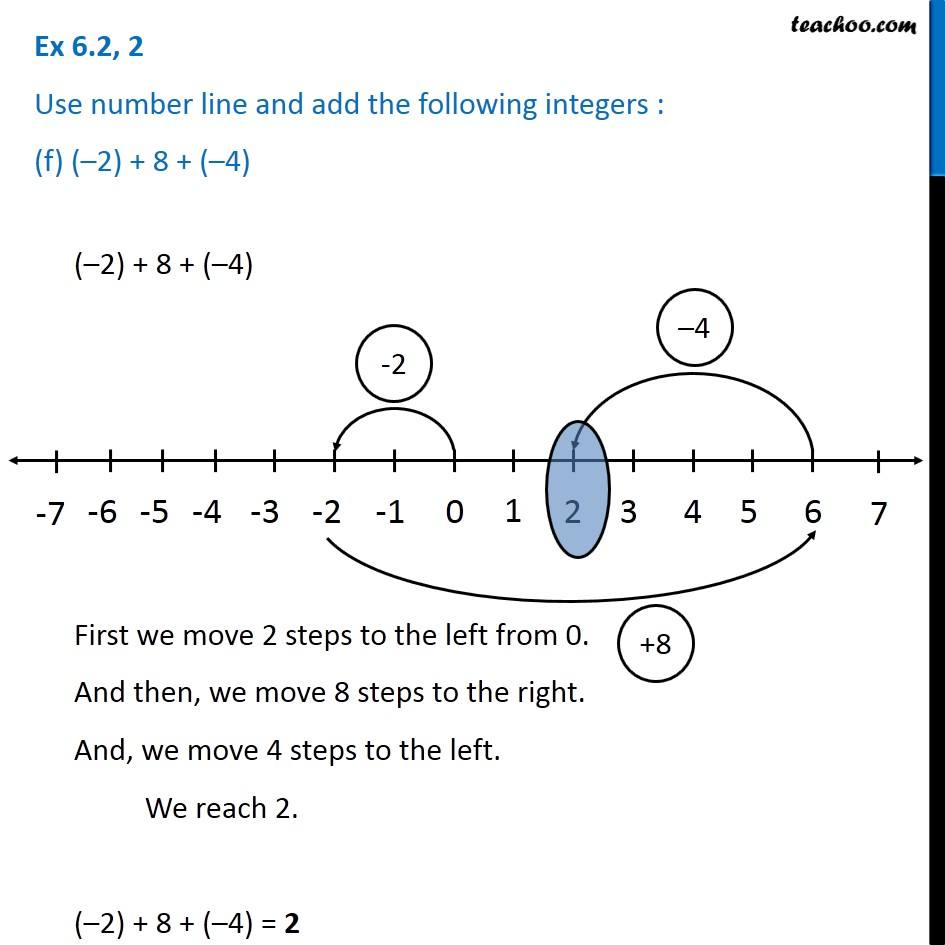Learn in your speed, with individual attention - Teachoo Maths 1-on-1 Class

### Transcript

Ex 6.2, 2 Use number line and add the following integers : (a) 9 + (– 6)9 + (–6) First we move 9 steps to the right from 0. And then, we move 6 steps to the left. We reach 3. ∴ 9 + (–6) = 3 Ex 6.2, 2 Use number line and add the following integers : (b) 5 + (– 11)5 + (–11) First we move 5 steps to the right from 0. And then, we move 11 steps to the left. We reach –6. ∴ 5 + (–11) = –6 Ex 6.2, 2 Use number line and add the following integers : (c) (– 1) + (– 7)(−1) + (–7) First we move 1 steps to the left from 0. And then, we move 7 steps to the left. We reach –8. ∴ (–1) + (7) = –8 Ex 6.2, 2 Use number line and add the following integers : (d) (– 5) + 10(–5) + 10 First we move 5 steps to the left from 0. And then, we move 10 steps to the right. We reach 5. ∴ (–5) + 10 = 5 Ex 6.2, 2 Use number line and add the following integers : (e) (– 1) + (– 2) + (– 3)(–1) + (–2) + (–3) First we move 1 steps to the left from 0. And then, we move 2 steps to the left. And, we move 3 steps to the left. We reach –6. ∴ (–1) + (–2) + (–3) = –6 Ex 6.2, 2 Use number line and add the following integers : (f) (–2) + 8 + (–4)(–2) + 8 + (–4) First we move 2 steps to the left from 0. And then, we move 8 steps to the right. And, we move 4 steps to the left. We reach 2. (–2) + 8 + (–4) = 2